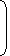# Tips and Tricks to simplify algebra problems1. (a + b)(a – b) = (a2 – b2)
2. (a + b)2 = (a2 + b2 + 2ab)
3. (a – b)2 = (a2 + b2 – 2ab)
4. (a + b + c)2 = a2 + b2 + c2 + 2(ab + bc + ca)
5. (a3 + b3) = (a + b)(a2 – ab + b2)
6. (a3 – b3) = (a – b)(a2 + ab + b2)
7. (a3 + b3 + c3 – 3abc) = (a + b + c)(a2 + b2 + c2 – ab – bc – ac)
8. When a + b + c = 0, then a3 + b3 + c3 = 3abc
Which one of the following is not a prime number?
 A. 31 B. 61 C. 71 D. 91

Explanation:

91 is divisible by 7. So, it is not a prime number.

2

(112 x 54) = ?
 A. 67000 B. 70000 C. 76500 D. 77200

Explanation:

 (112 x 54) = 112 x104 = 112 x 104 = 1120000 = 70000 2 24 16
9. #### It is being given that (232 + 1) is completely divisible by a whole number. Which of the following numbers is completely divisible by this number?

Correct! Wrong!

#### What least number must be added to 1056, so that the sum is completely divisible by 23 ?

Correct! Wrong!

#### 1397 x 1397 = ?

Correct! Wrong!

#### How many of the following numbers are divisible by 132 ? 264, 396, 462, 792, 968, 2178, 5184, 6336

Correct! Wrong!

#### Which of the following is a prime number ?

Correct! Wrong!

QUIZ ON NUMBERS
WELL DONE !!!